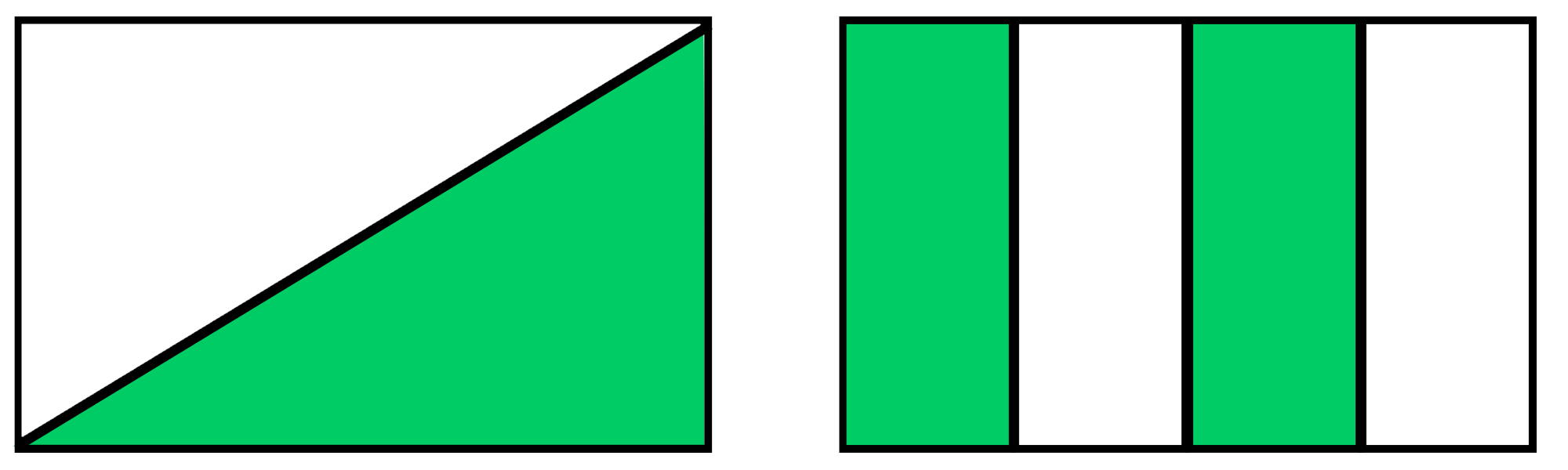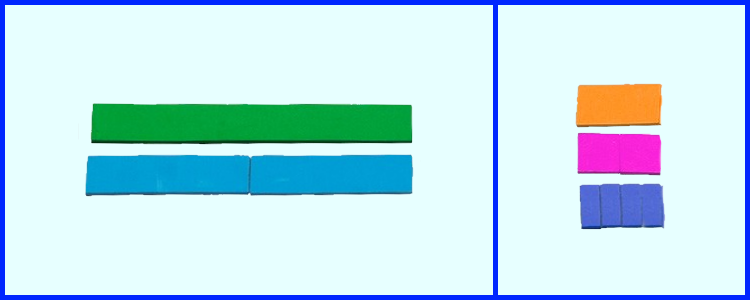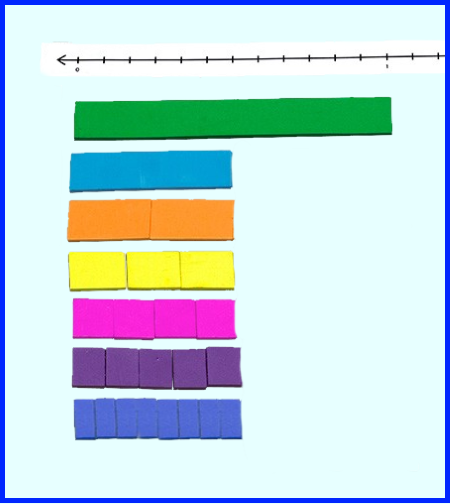By: Kit Norris

As I travel around the country working with teachers, I frequently hear, “My students just don’t like fractions.” Teachers are right, fractions are confusing. Let’s explore the foundational understandings and possible misconceptions that students may go through as they are learning about fractions.

As articulated in the progression on “Number and Operations-Fractions, 3-5,” fractional understanding begins in Grades 1 and 2 as students partition shapes. This is certainly a logical beginning as students have had experiences identifying when their share is smaller than someone else’s!  In Grade 3, students begin considering breaking a whole into equal parts.  Students work with wholes that are varying shapes such as rectangles or circles  and the focus is placed on equal parts.  The emphasis in Grade 3 is on unit fractions (i.e., fractions having 1 as the numerator). Just as our whole numbers are composed by combining 1s, fractions can be similarly constructed by combining unit fractions. For example, ¾ = ¼ + ¼ + ¼.

This seems reasonably straightforward at this point. So, what might a student be thinking when considering ½? What are the possible misconceptions a student might be thinking about?

Students’ early awareness of half is associated with sharing and fairness. Students might say “I got half” or “We all got half.” Students may associate half with two parts regardless of the size of each of the parts. Students need experiences to realize that the two parts may not even appear to look alike, but they must have the same quantity.Fraction notation can also cause confusion for some students who do not associate any meaning to the two numbers used to represent a fraction. They simply see a 1 and a 2 and say “the two is bigger than 1.”

Watching for these early misconceptions can help pave the way for solid understanding as students continue their learning about fractions.

Fraction strips are a simple tool to develop conceptual understanding of fractions. The fraction strips are color-coded and they are not marked in any other way. The reason for this is that we want students to determine size of the parts by comparing them to the whole rather than simply reading a label.

The following activities provide a beginning framework for using these fraction strips in order to build students’ conceptual understanding. As students are working with the strips, teachers are posing questions and listening to students’ responses as they are making sense of their work and justifying their conclusions.

Understanding the Whole

Start by placing the dark green strip on board. Say, “This is one whole. What size is the light blue strip? How do you know?” Repeat asking about other colors in reference to the whole. Now show the number line. Ask students to place different size strips on the number line using the dark green as the whole. This can be placed above the number line for reference. Teachers should label the number line to indicate the whole using 0 and 1.Now, ask students to determine the number of unit fractions needed to make a whole using the light blue strip.  Ask, “How many of these strips are the same as the whole? How many strips the size of ⅛ make the whole?” Ask students to count these pieces as they are placed on the number line.

Repeat the activity using the orange strip as the whole. Ask, “What is the size of the magenta strip? The dark blue strip? How do you know?” Have students use the number line to explore these relationships. Summarize the activity by having a discussion about why the strips changed their value, and what caused the change.

Equivalence

Show the green strip. Say, “The green represents one whole. How many ways can you represent ½?” Ask students to write the equivalent fractions and compare their list with a partner.

Continue the activity by asking students to find all of the fractions that are equivalent to ¼ and ⅛. Extend students’ thinking by asking them to represent the equivalent fractions in terms of a number sentence using only unit fractions. For example, ½ = ¼ + ¼ or ½ = ⅙ + ⅙ + ⅙.Using the fraction strips, students can build their conceptual understanding of fractions by beginning with the representation of the whole and working to interpret the other fractions in relation to the whole.

Kit Norris is a former mathematics educator, administrator, and supervisor who works with teachers and administrators to implement best practices in math. An award-winning educator, consultant, and author, Kit was inducted into the Mathematics Educators Hall of Fame in Massachusetts in 2015. She is a frequent speaker at national, regional, and local conferences and has served on the board of directors of the National Council of Supervisors of Mathematics. Kit has developed several products with Didax, including the Magnetic Fraction Number Line and Fraction Tile Number Line sets.